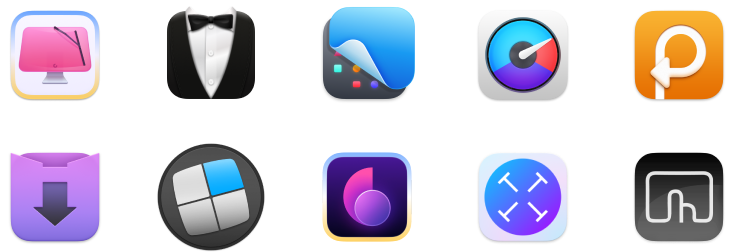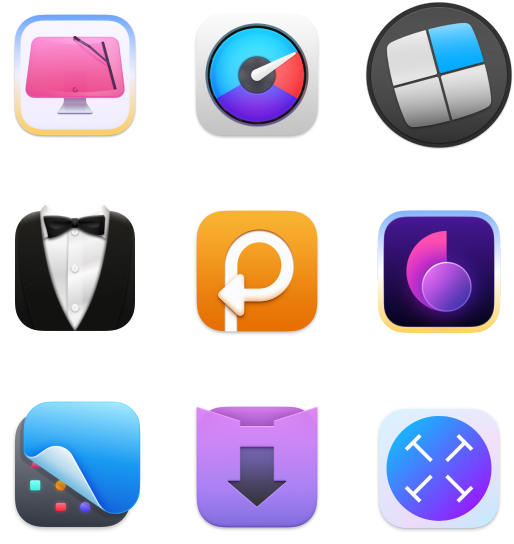# How to calculate percentages

17.2K views

You probably learned and used the percentage formula many years ago. It’s essentially a way to represent a ratio as a number. So instead of something like “44/100,” you could just say “44 percent.”

Percentages have tons of use in our daily lives. We use it to discuss how close we are to finishing projects, our confidence level in something, or how much of a given goal we’ve achieved so far. In this article, we’ll discuss how to calculate percentages, and surface some really handy percentage calculator apps for Mac we know you’ll love.

## How to Use Percentage Calculator

We’d venture to say most of the time you need to reference percentages, it’s to find a percentage of something. We’ll also go out on a limb and say you probably don’t want to mess around with a calculator every time you want to know a percentage.

Let’s be honest: math isn’t always easy. Even the simplest mathematical operations slip by the sharpest minds. Most of us will break out a calculator for even simple math, but how many times have you used the calculator to qualify even the most basic calculations?

Now think about how many times those results were obviously wrong because your calculator had some number in its immediate memory from an older calculation. What if you never caught it?!

That’s why we like Numi. Your brain isn’t a calculator, and Numi bridges the gap between language and numbers with ease. It allows you to find percentages of numbers and do things like add percentages to numbers without a ton of wonky math.

Its documentation shows you with ease how to find percentage of something without a ton of steps. Here’s the breakdown:

• Percentage value 20% of \$10
• Adding percentage 5% on \$30
• Subtracting percentage 6% off 40 EUR
• Percentage value of one value relative to another \$50 as a % of \$100
• Percentage addition of one value relative to another \$70 as a % on \$20
• Percentage subtraction of one value relative to another \$20 as a % off \$70
• Value by percent part 5% of what is 6 EUR
• Value by percent addition 5% on what is 6 EUR
• Value by percent subtraction 5% off what is 6 EUR

Let’s walk through a simple exercise. What if your bill came to \$33, and you wanted to add five percent onto it because you really wanted to support the person who made whatever you’re buying. Here’s how to use Numi to quickly figure out what five percent of \$33 is, and what the total of your bill would be if you added the five percent onto it:

1. Open Numi
2. The first screen you’ll see is the ‘sample’ screen. Press the ‘plus’ icon on the top right of the screen to open a new calculations window
3. Enter the percentages or figures you’d like to figure out. Here we’ll ask what five percent of \$33 is, and add it onto our \$33 bill.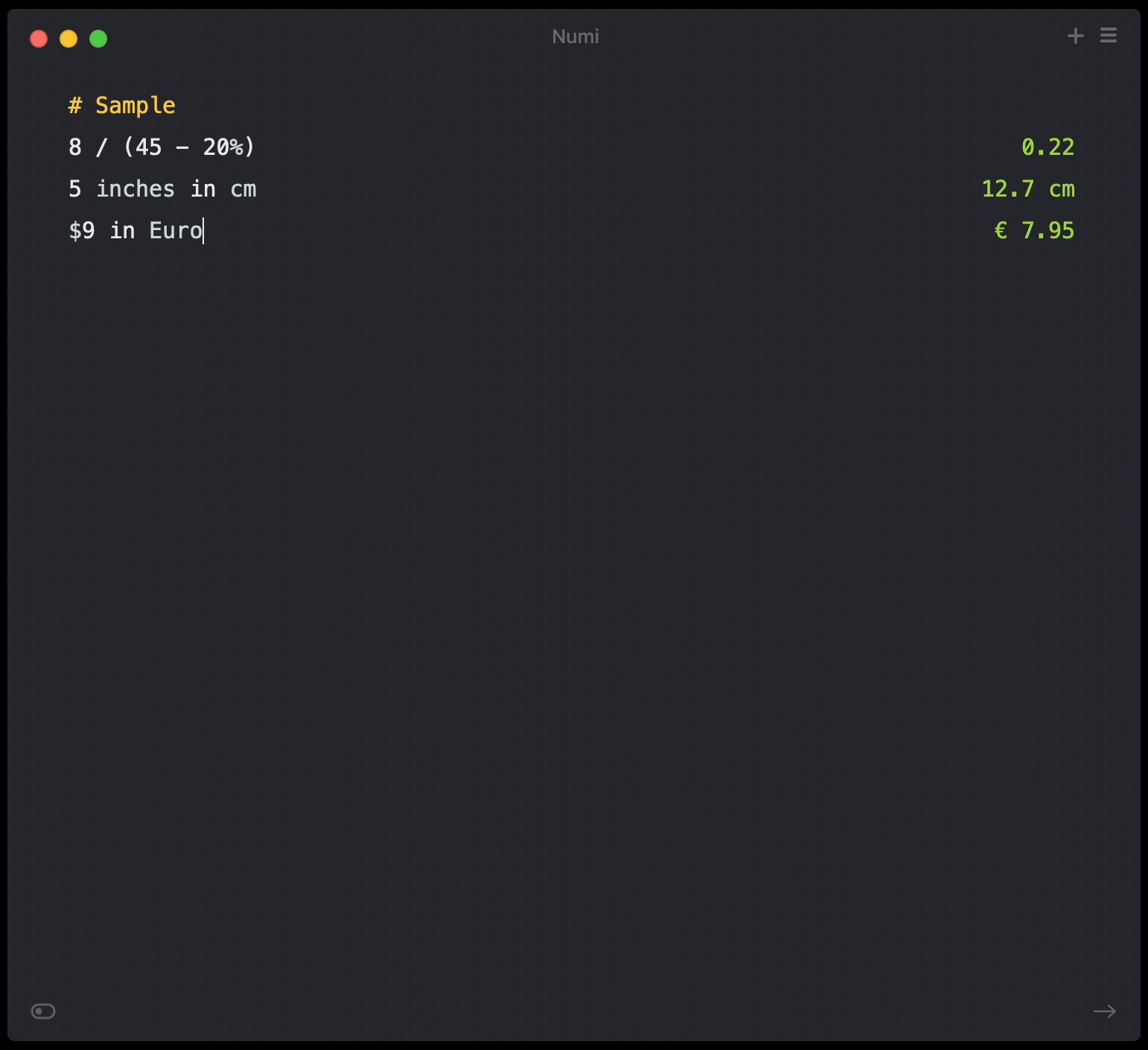Here’s the best part: we didn’t even need the first command! Numi is adept at natural language for mathematical purposes, so we could have simply entered “\$33 add 5%” and seen the total bill. And let’s be honest: that’s what matters to your bank account, right?!

## How to Calculate Percentage of a Whole

When you want to know how to find percentage for a whole, two things matter: the part, and the whole.

Let’s run through another exercise. A developer is working on a new website, and has coded 4,413 lines. Of those 4,413 lines, 741 have errors that need to be resolved. This developer wants to know how ‘right’ they were on the first pass at coding this site, and thinks it may be fun to know what percentage of their code was buggy as they coded.

Here’s how to find out the old fashioned way:

1. Discover the whole. Here we know it’s 4,413
2. Discover the part. Here we know it’s 741
3. Divide the part by the whole: 741/4,413
4. View the result: 0.1679.
We know that can’t be right, so now what? The easiest way to transfer this to a percentage is to slide the decimal point two spots right. Remember a percentage is always a representation of a whole. Regardless of the number, the ‘whole’ is always 100 percent. (We like to remember sliding two spots to the left as the two zeros in ‘100’.)
5. Slide the decimal point to find the correct percentage: 16.79 percent

So, our developer friend has 16.79 percent buggy code. We’re sure fixing one line will spring several more errors so best of luck, friend!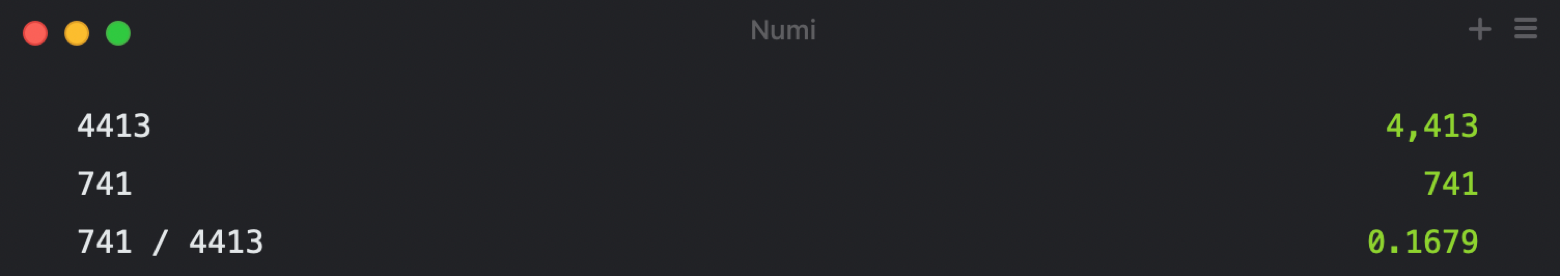## How to Calculate Percentage Working Backwards

Working backwards has its own formula: X of Y is Z.

Here’s how it all works. Again, an exercise: let’s say you borrowed money from a friend, and they don’t trust you to pay them back in a reasonable time. So they decide to charge you five percent every week you don’t pay them back.

Here’s how to calculate your first month’s interest, and how much it would cost you if you didn’t pay them back within a month:

• ‘Y’ is the total amount borrowed: \$300
• ‘X’ is the interest: five percent
• ‘Z’ is Y times X, and the result added to Y
1. Divide five by 100: 0.05 - this is the amount per dollar borrowed you owe in interest
2. Multiply 0.05 by \$300: \$15
3. Add \$15 to \$300: \$315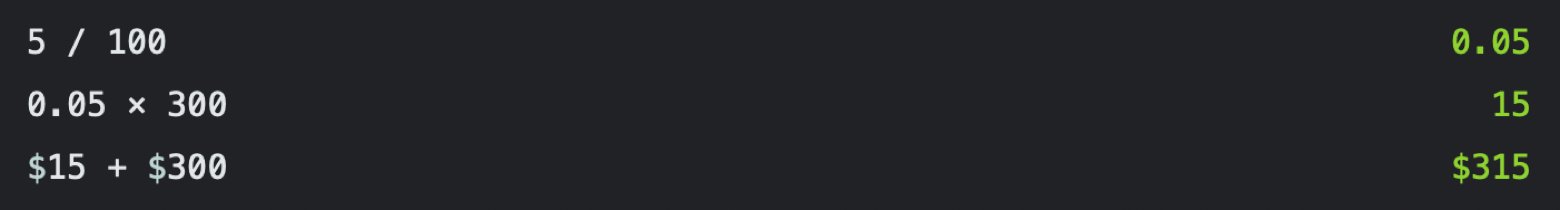## Is There a Percentage Formula

You can also calculate percentages in Excel or Google Sheets, but know these are most accurate in showing a percent change between two cells. You can also find the percentage of a number, but it has to be hard-coded into a cell. As an example, let's say we need to find what 30 percent of 584 is. In a cell, we can enter the following:

=30%*584

The cell will show “175.2” which is correct. 175.2 is 30 percent of 584. You can also use this equals-first formula to calculate the percentage of two numbers. Let’s say you needed to know what percentage of 584 the number 175.2 was. You could just enter the following to see the number “0.3” in the cell:

=175.2/584

If you wanted to view it as a percent, simply format the cell to show percent figures.

If you’re going to use Excel, check out our article on setting up and using the Microsoft Office Suite for Mac. It has everything you’ll need to know to get up and running with Excel!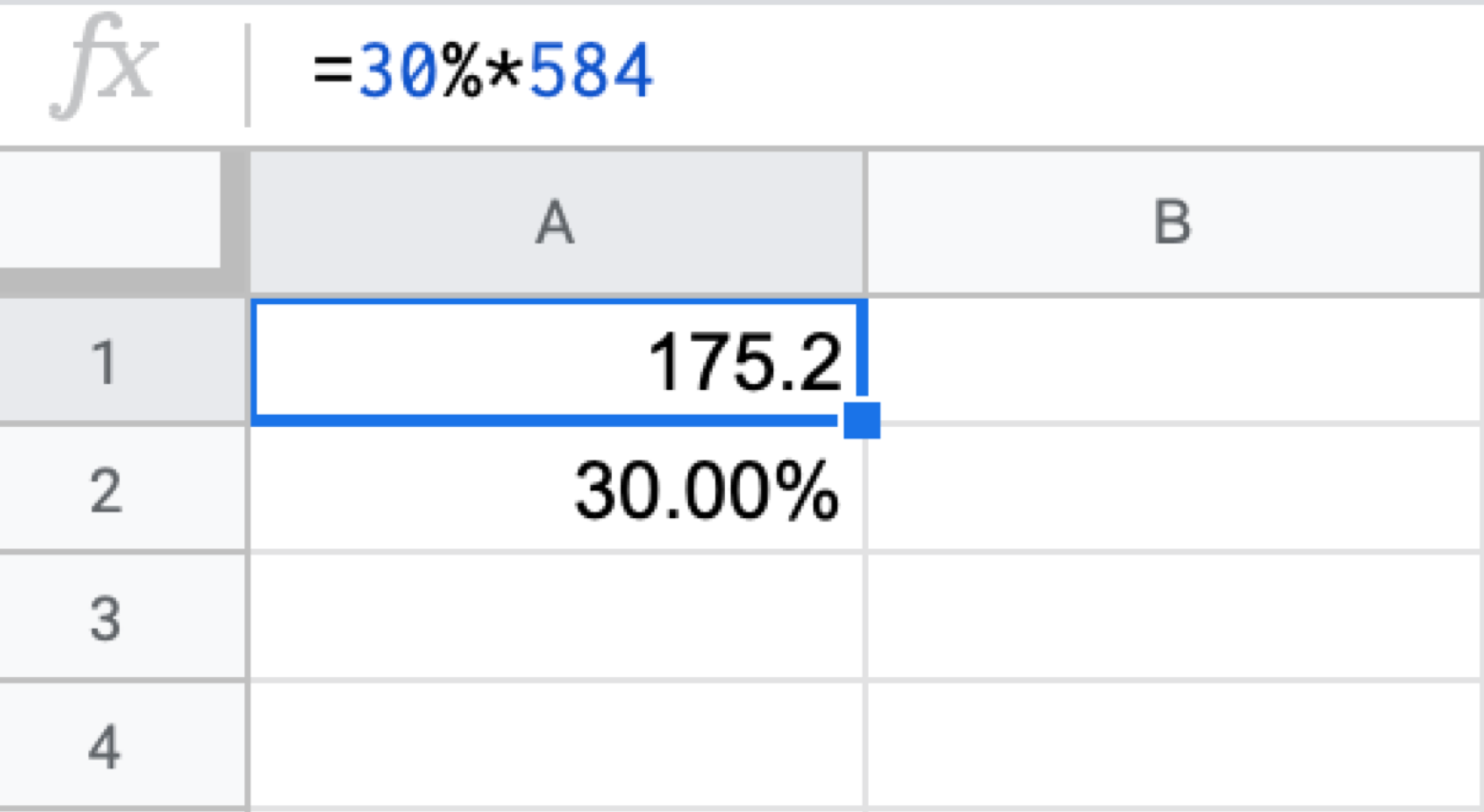## How to Do Percentages to Calculate Discounts

Another common use for percentages is calculating discounts. You’ve likely seen offers for receiving a discount on an item, but no final cost. Here’s how to calculate your discount:

1. Know the original price. Let’s say it’s a \$90 item
2. Discover the discount. We’ll say 20 percent, here
3. Find out the final cost as a percentage by subtracting 20 percent from 100 percent: 100 - 20 = 80
4. Divide 80 by 100: 80/100 = 0.8
5. Multiply the price of your item by 0.8: 90 x 0.8 = 72

If you’re shopping often, it’s probably a good idea to track your spending with an app like MoneyWiz. The app links to all of your credit cards and bank accounts to help you track finances without dipping and diving between web pages. It’s a really sharp way to track spending and know whether a promised discount was actually applied. Often your discount code is meant to be applied later, but never actually is. MoneyWiz can help you track those things.

It’s also great for budgeting. If you needed to manage spending in a category, like clothing, MoneyWiz will help you know exactly how much of your annual budget that cool new jacket is.

### Conclusion

Percentages aren’t always easy. If we’re being clever, we will note all the long-hand percentages we’ve shown you in this article are one-line commands in Numi. That’s why we really like it; no more fussing with long form math. Just ask Numi, and it’ll tell you the answer!

MoneyWiz and Numi are also free as part of a seven day trial of Setapp, the world’s best suite of productivity apps for the Mac. You’ll also have immediate and unlimited access to nearly 200 other apps across a wide range of uses from email to coding to – well, almost anything!

When your trial is over, Setapp is only \$9.99 per month for unlimited access. If you decide to pre-pay for a full year, that price drops ten percent to \$8.99 per month. (Okay, really 10.01 percent. Thanks, Numi!)

Families with multiple Macs will love Setapp’s family plan, a \$19.99 per month subscription that earns you access to the full Setapp catalog on up to four Macs. Give Setapp a try today! We know you’ll love it.240+ apps for \$9.99 per monthSecurity-tested